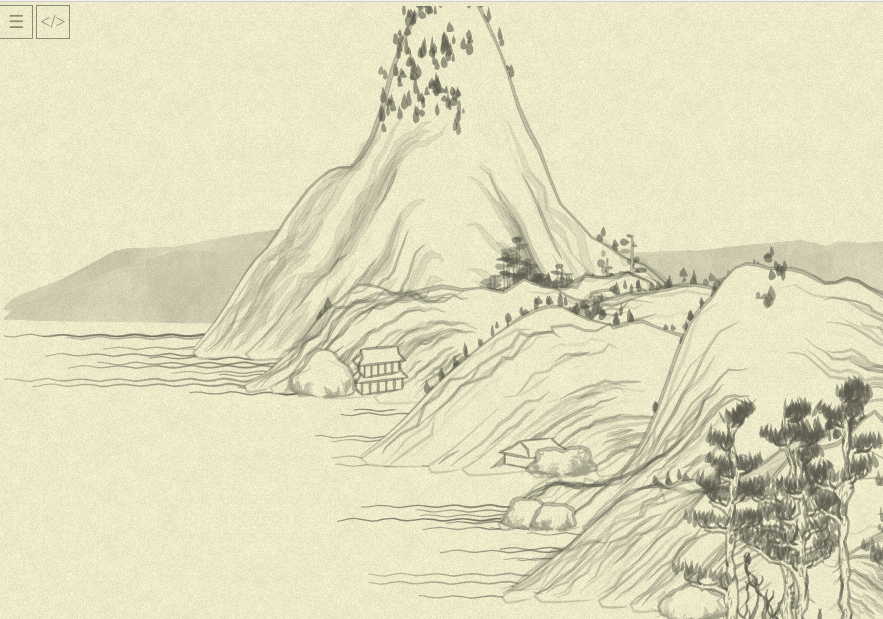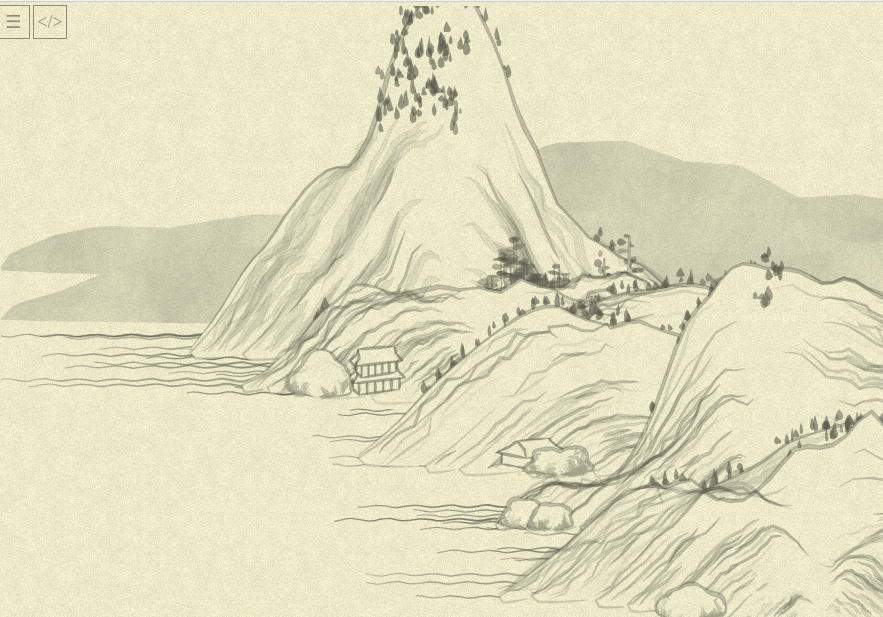« Day 12 | Day 14 »

• Commit: 297259f
• Functions:
• `mountplanner`: 1314

So, let’s crack that `mountplanner` thing!

Random thing to spend time on: `rand(230, 280)` is NOT the same as `280 - rand(50)` at the same step of the random generator, while looking … logically the same?… Why?.. (10 min debug). Oh, because while `Math.random()` gives exactly the same value on the same step… `rand(from, to)` would be the same as `230 + rand(50)`, which is NOT the same at all (just replace `rand(50)` with some particular value to check). So, while it is “logically nice to see” `y: rand(230, 280)` where `y: 280 - Math.random() * 50` was, we can’t allow that!

Today would be word-low day :) After some time, I managed to mechanically simplify the main function’s body to this:

``````var x_range = range(xmin, xmax, xstep)

x_range.forEach( x => MEM.planmtx[Math.floor(x / xstep)] ||= 0)

x_range.forEach( x => {
var max_y = yr(x) * 480
range(0, max_y, 30).forEach( y => {
if (locmax(x, y, ns, 2)) {
var x_offset = x + rand(-500, 500);
var y_offset = y + 300;
if (chadd({ tag: "mount", x: x_offset, y: y_offset, h: ns(x, y) })) {
range(Math.floor((x_offset - mwid) / xstep), (x_offset + mwid) / xstep).
forEach( dx => MEM.planmtx[dx] += 1 )
}
}
})
if (Math.abs(x) % 1000 < Math.max(1, xstep - 1)) {
tag: "distmount",
x: x,
y: 280 - rand(50),
h: ns(x, max_y),
});
}
})

x_range.forEach( x => {
if (MEM.planmtx[Math.floor(x / xstep)] == 0) {
chance(0.01, () => {
for (var y = 0; y < rand(4); y++) {
tag: "flatmount",
x: x + rand(-700, 700),
y: 700 - y * 50,
h: ns(x, y),
});
}
})
}
})

x_range.forEach( x =>
chance(0.2, () => chadd({ tag: "boat", x, y: 300 + rand(390) }, 400))
)
``````

(…as usually, I went through several hoops of “why the heck it does this now?..” but actually much lower amount than I’ve expected, and they all were trivial/seen before, so I am skipping this part.)

This new form clarifies things a bit (for me! — will I every became tired of adding this clarification?.. please imagine it everywhere), due to pure squashing of details into higher-level atoms. I enhanced our `range` function (which previously could only `range(max)`) to this:

``````// range(4) => [0, 1, 2, 3]
// range(1, 4) => [1, 2, 3]
// range(1, 10, 2) => [1, 3, 5, 7, 9]
function range(from, to = undefined, step = undefined) {
if(to == undefined) {
to = from
from = 0
}
step ||= 1
var res = []
for(i = from; i < to; i += step) {
res.push(i)
}
return res;
}
``````

Funny note: here I am rather mimicking Python than Ruby (Ruby-idiomatic way to say “range with begin, end, and step” would be `(1...4).step(2)` or `(1...4) % 2`. This is much more work to reproduce—and without a dedicated range literal Ruby has, this will still be awkward.)

There are a few more things to (slightly) clarify—more or less it looks ready-for-explanation now.

Another thing to probably notice is all cycles are replaced with `range(...).forEach` except for one:

``````for (var y = 0; y < rand(4); y++) {
tag: "flatmount",
x: x + rand(-700, 700),
y: 700 - y * 50,
h: ns(x, y),
});
}
``````

It cost me a dear 10 minutes of head-scratching and debugging: once you replace it with (natural, I thought, eh?) this:

``````range(rand(4)).forEach( y => {
tag: "flatmount",
x: x + rand(-700, 700),
y: 700 - y * 50,
h: ns(x, y),
});
})
``````

…the picture is suddenly screwed.

Right:With cool range (notice the background mountains shift, and foreground trees vanishing):…only after extracting “cycle end” to a variable for debugging I noticed/remembered my C days, and that cycle condition is calculated on every cycle run: so where with `range` we have exactly one call to `random()`, “classic” cycle re-randoms every iteration. It was probably not intended by the original author, but for the sake of preserving the exact picture I put the cycle back.

So… that’s that for today.

Tomorrow I want to tackle some of the naming, internal methods like `locmax` (which is obviously `local_maximum`, so maybe just leave it be?) and the interaction with `MEM.planmtx`. It is, again, not too murky (global storage of “already taken” squares of the picture), but maybe it can be more high-level, too?

« Day 12 | Day 14 »